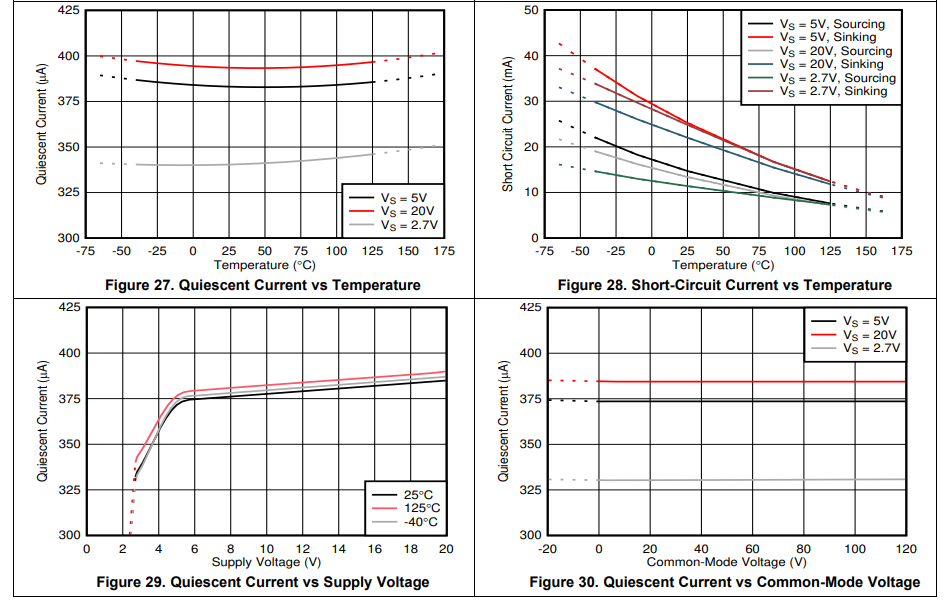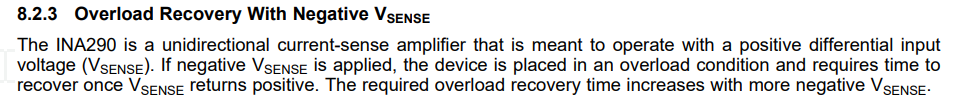If you have a related question, please click the "Ask a related question" button in the top right corner. The newly created question will be automatically linked to this question.

INA290: How can I read current near to zero?

Part Number: INA290

The output voltage of INA290 is 0.025V which leave us blind when reading low currents. do you have any suggestion to solve such problem.

• Use a larger sense resistor.

(This is why many multimeters require you to select a range.)

• Yesaiahu,

When measuring low currents one needs to take into account the VOS, swing low, and IB of the input of a current sense amplifier.  As pointed out increasing the Rshunt value will increase the value of you input but you will give up power dissipation at higher currents.  If low current measurements down to zero are required then you may need to look for a bidirectional device, as the INA290 is unidirectional, and place a voltage at the reference pin above ground to get measurements at zero current.  In this case you zero current output voltage will equal to your Vref voltage.

Impacts on Measurement from current sense amplifiers.

VOS -->  This will impact your lowest input voltage measured.  If Vos =12µV; your error with a gain at 500 = 12µ*500 =6mV  (This will not be your limiting factor but explaining the limitation for other devices.

Swing low -->  This is the lowest the output can get to GND.  So if VOS is not dominating when placing 0V at the input then this is the minimum you can measure.

IB  -->  In very low current applications when measuring in µA the input bias current will play a bid deal in the error in the measurement.  For this device the IB is in the 30µA range.

• Thank you Javier for the answer.
The problem remains. I need near 0 reading.

May be a TI expert can elaborate other methods like: Adding a 100 ohm resistor between the GND leg and the circuit ground.
this simple trik may solve the problem but I need some details like:
1- Operating current vs temperature change.
2- Operating current vs to common- mode voltage.
and so on.
The quiescent operating current impact can be easily calibrate by software.

• Hello Yesaiahu,

The output is limited but this will not change that limit on the input stage side. Like I mentioned if you would want more accurate number you would need to use a bidirectional device with a Vref pin so you can control the output voltage when the input is zero.

You could change the input and add a resistor and use the input bias current to your advantage but I would not recommend that as it will add both Vos, gain error and Common mode rejection error.

We have provided two of the three plots you ask for in the datasheet.  Since you are considering the low input please be aware about the bandwidth limitations as seen in the datasheet as well as when you go below 0V at the input that the INA290 does take some time to recover.  I have attached screen shots below.